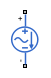# Sinusoidal Current Source

Damped sinusoidal current source

•Libraries:
Simscape / Electrical / Additional Components / SPICE Sources

## Description

The Sinusoidal Current Source block represents a damped sinusoidal current source whose output current is independent of the voltage across the terminals of the source. The following equations describe the current through the source as a function of time:

`$\begin{array}{l}{I}_{out}\left(Time\le TD\right)=IO\\ {I}_{out}\left(Time>TD\right)=IO+IA*{e}^{-\left(Time-TD\right)*DF}*\mathrm{sin}\left(2\pi *FREQ*\left(Time-TD\right)\right)\end{array}$`

where:

• I0 is the Current offset, IO parameter value.

• IA is the Sinusoidal amplitude, IA parameter value.

• FREQ is the Sinusoidal frequency, FREQ parameter value.

• TD is the Time delay, TD parameter value.

• DF is the Damping factor, DF parameter value.

The block uses a small conductance internally to prevent numerical simulation issues. The conductance connects the + and - ports of the device and has a conductance GMIN:

• By default, GMIN matches the GMIN parameter of the Environment Parameters block, whose default value is `1e–12`.

• To change GMIN, add an Environment Parameters block to your model and set the GMIN parameter to the desired value.

## Ports

### Conserving

expand all

Electrical conserving port associated with the sinusoidal current source positive terminal.

Electrical conserving port associated with the sinusoidal current source negative terminal.

## Parameters

expand all

The magnitude of the time-independent part of the output current.

The magnitude of the sinusoidal part of the output current.

The frequency of the output sine wave. The value can be less than `0`.

The time at which the sine wave first starts. A value less than `0` indicates a phase shift of 180 degrees.

The exponential damping factor for the sine wave to produce the output current. If DF is greater than or equal to `0`, the exponential part of the equation is always damping for time greater than TD. The value must be greater than or equal to `0`.

Specify if the current source includes a parallel conductance. The value of the parallel conductance, GMIN, depends on whether your model contains an Environment Parameters block.

This table shows the possible values of the parallel conductance, GMIN:

Values of parallel conductance, GMIN

Include parallel conductance, GMIN
`Yes``No`
Model contains Environment Parameters blockModel does not contain Environment Parameters blockParallel conductance, GMIN, is not included and it does not affect the current source.
GMIN is equal to the value of the GMIN parameter in the Environment Parameters block.GMIN = `1e-12` `Ohm`

## Version History

Introduced in R2008a